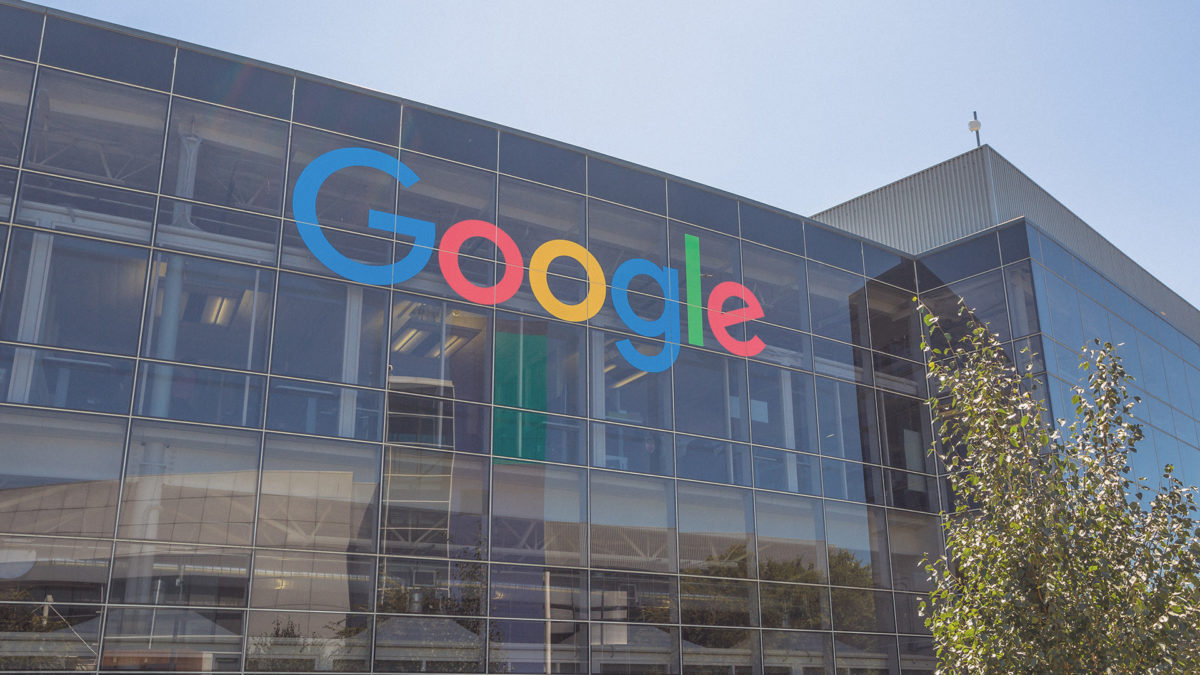# Google claims it's quantum computer can perform a task beyond the reach of any classical supercomputerIn a paper published on Wednesday, Google researchers claimed its quantum computer can, for the first time, perform a task "beyond the reach of the fastest classical supercomputers."

Google said it has devised a 53-bit quantum computer, called Sycamore, to compute a mathematical problem within 200 seconds. The same problem would take the world's fastest supercomputers over 10,000 years to solve, according to the paper.

The mathematical problem essentially asks computers to calculate the probability of a string of random numbers to verify its randomness. The paper notes that Sycamore's ability to complete the problem quickly makes it useful at generating certifiable random numbers, optimization, machine learning, materials science, and chemistry.

While normal computers store information in bits that represent either 1 or 0, quantum computers use quantum bits, or qubits, that can be both 1 and 0 at the same time. This so-called "superposition" state is what allows quantum computers to achieve the speed that's beyond the reach of classical computers.

However, for now, Google's quantum computer is still not able to run well-known quantum algorithms such as Shor's algorithm, which, if realized, could pose substantial threats to the existing public-key encryption scheme.

In a Monday blog post, IBM said that the mathematical problem Google used in fact only takes a classical supercomputer 2.5 days to solve if different techniques are adopted.